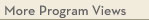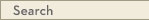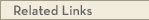# University Catalogs

Duluth campusDuluth Campus

# Statistics and Actuarial Science B.S.

Mathematics & Statistics
Swenson College of Science and Engineering
• Program Type: Baccalaureate
• Requirements for this program are current for Spring 2021
• Required credits to graduate with this degree: 120
• Required credits within the major: 56 to 57
• Degree: Bachelor of Science
The science of statistics is concerned with generating and analyzing data. Actuarial science applies statistical methods to assess risk in the insurance and financial industries. The statistics and actuarial science major prepares students for careers in a wide variety of fields, from banking and government to health care. Advisors have information on the national actuarial examinations. Honors Requirements: To graduate with department honors, a student must complete the program with an overall and department GPA of 3.50, satisfactorily complete a research project under the guidance of a faculty member, and convey research results in a public presentation.
Program Delivery
This program is available:
• via classroom (the majority of instruction is face-to-face)
General Requirements
The Board of Regents, on recommendation of the faculty, grants degrees from the University of Minnesota. Requirements for an undergraduate degree from University of Minnesota Duluth include the following:
1. Students must meet all course and credit requirements of the departments and colleges or schools in which they are enrolled including an advanced writing course. Students seeking two degrees must fulfill the requirements of both degrees. However, two degrees cannot be awarded for the same major.
2. Students must complete all requirements of the Liberal Education Program.
3. Students must complete a minimum of 120 semester credits.
4. At least 30 semester credits must be earned through UMD, and 15 of the last 30 degree credits earned immediately before graduation must be awarded by UMD.
5. Students must complete at least half of their courses at the 3xxx-level and higher at UMD. Study-abroad credits earned through courses taught by UM faculty and at institutions with which UMD has international exchange programs may be used to fulfill this requirement.
6. If a minor is required, students must take at least three upper division credits in their minor field from UMD.
7. For certificate programs, at least 3 upper-division credits that satisfy requirements for the certificate must be taken through UMD. If the program does not require upper division credits students must take at least one course from the certificate program from UMD.
8. The minimum cumulative UM GPA required for graduation will be 2.00 and will include only University of Minnesota coursework. A minimum UM GPA of 2.00 is required in each UMD undergraduate major and minor. No academic unit may impose higher grade point standards to graduate.
9. Diploma, transcripts, and certification will be withheld until all financial obligations to the University have been met.
Program Requirements
1. A minor or a second major from another department.
Calculus Courses (10 cr)
Calculus I
Take one of the following Calculus I courses:
MATH 1290 - Calculus for the Natural Sciences [LE CAT2, LOGIC & QR] (5.0 cr)
or MATH 1296 - Calculus I [LE CAT, LOGIC & QR] (5.0 cr)
Calculus II
MATH 1297 - Calculus II [LOGIC & QR] (5.0 cr)
Statistics and Actuarial Science Core Courses (42-43 cr)
Mathematics Core I
MATH 3280 - Differential Equations with Linear Algebra (4.0 cr)
MATH 3298 - Calculus III (4.0 cr)
MATH 3355 - Discrete Mathematics (4.0 cr)
Mathematics Core II
MATH 4201 - Elementary Real Analysis (4.0 cr)
or MATH 4326 - Linear Algebra (3.0 cr)
Statistics Core I
STAT 3611 - Introduction to Probability and Statistics (4.0 cr)
STAT 3612 - Introduction to Probability and Statistics II (3.0 cr)
Statistics Core II
STAT 5511 - Regression Analysis (3.0 cr)
STAT 5531 - Probability Models (4.0 cr)
STAT 5571 - Probability (4.0 cr)
STAT 5572 - Statistical Inference (4.0 cr)
Computer Science
CS 1511 - Computer Science I [LE CAT, LOGIC & QR] (5.0 cr)
or CS 1581 - Honors: Computer Science I [LE CAT3, LOGIC & QR] (5.0 cr)
Required Math Undergraduate Colloquium (1 cr)
Students must register their last semester before graduation.
MATH 3941 - Undergraduate Colloquium (1.0 cr)View college catalog(s): · Swenson College of Science and Engineering View sample plan(s): · Statistics and Actuarial Science BS View checkpoint chart: · Statistics and Actuarial Science B.S. View PDF Version:Search Programs Search University CatalogsOne Stop for tuition, course registration, financial aid, academic calendars, and moreMATH 1290 - Calculus for the Natural Sciences (LE CAT2, LOGIC & QR)
 Credits: 5.0 [max 5.0] Course Equivalencies: 01420 - Math1290/1296/1596 Grading Basis: A-F or Aud Typically offered: Every Fall & Spring
Differential and integral calculus needed for modeling in earth and life sciences. Computational software. Not intended for students in mathematics, engineering, or physical sciences. prereq: Math ACT 27 or higher or a grade of at least C- in Math 1250 or department consent
MATH 1296 - Calculus I (LE CAT, LOGIC & QR)
 Credits: 5.0 [max 5.0] Course Equivalencies: 01420 Grading Basis: A-F or Aud Typically offered: Every Fall, Spring & Summer
First part of a standard introduction to calculus of functions of a single variable. Limits, continuity, derivatives, integrals, and their applications. prereq: Math ACT 27 or higher or a grade of at least C- in Math 1250 or department consent
MATH 1297 - Calculus II (LOGIC & QR)
 Credits: 5.0 [max 5.0] Course Equivalencies: 01421 Grading Basis: A-F or Aud Typically offered: Every Fall, Spring & Summer
Second part of a standard introduction to calculus. Vectors, applications of integrals, transcendental functions, series, and multivariable functions and partial derivatives. prereq: A grade of at least C- in 1290 or 1296 or 1596
MATH 3280 - Differential Equations with Linear Algebra
 Credits: 4.0 [max 4.0] Grading Basis: A-F or Aud Typically offered: Every Fall, Spring & Summer
First, second, and higher order equations; series methods; Laplace transforms; systems; software; modeling applications; introduction to vectors; matrix algebra, eigenvalues. prereq: A grade of at least C- in 1297 or 1597
MATH 3298 - Calculus III
 Credits: 4.0 [max 4.0] Grading Basis: A-F or Aud Typically offered: Every Fall, Spring & Summer
Third part of a standard introduction to calculus. Conic sections, vectors and vector-valued functions, partial derivatives and multiple integrals, vector fields, Green's and Stokes' theorems. prereq: A grade of at least C- in 1297 or 1597
MATH 3355 - Discrete Mathematics
 Credits: 4.0 [max 4.0] Grading Basis: A-F or Aud Typically offered: Every Fall & Spring
Introduction to mathematical logic, predicates and quantifiers, sets, proof techniques, recursion and mathematical induction, recursive algorithms, analysis of algorithms, assertions and loop invariants, complexity measures of algorithms, combinatorial counting techniques, relations, graph theory. prereq: 1297 or 1597 or instructor consent, a grade of C- or better in is required in all prerequisite courses
MATH 4201 - Elementary Real Analysis
 Credits: 4.0 [max 4.0] Grading Basis: A-F or Aud Typically offered: Every Fall & Spring
In-depth study of concepts fundamental to the theory of single-variable calculus, including topology of the real numbers, convergence of sequences and series, function continuity, the derivative, and the Riemann integral. prereq: 3280, 3355, a grade of C- or better in is required in all prerequisite courses, no grad credit; credit will not be granted if already received for 3299
MATH 4326 - Linear Algebra
 Credits: 3.0 [max 3.0] Grading Basis: A-F or Aud Typically offered: Every Fall & Spring
Systems of linear equations, matrix algebra, determinants, vector spaces, subspaces, linear independence, span, basis, coordinates, linear transformations, matrix representations of linear transformations, eigenvalues and eigenvectors, diagonalization, Gram-Schmidt orthogonalization, orthogonal projection and least squares. prereq: A grade of at least C- in 3280, 3355, no grad credit
STAT 3611 - Introduction to Probability and Statistics
 Credits: 4.0 [max 4.0] Grading Basis: A-F or Aud Typically offered: Every Fall, Spring & Summer
Basic probability, including combinatorial methods, random variables, mathematical expectation. Binomial, normal, and other standard distributions. Moment-generating functions. Basic statistics, including descriptive statistics and sampling distributions. Estimation and statistical hypothesis testing. prereq: A grade of at least C- in Math 1290 or Math 1296
STAT 3612 - Introduction to Probability and Statistics II
 Credits: 3.0 [max 3.0] Grading Basis: A-F or Aud Typically offered: Every Fall & Spring
An introduction to statistics. Sample distributions, point and interval estimation, hypothesis testing, linear regression, one- and two-way analysis of variance, goodness-of-fit and non-parametric statistics. prereq: 3611 and Math 1297 or equivalent or instructor consent
STAT 5511 - Regression Analysis
 Credits: 3.0 [max 3.0] Prerequisites: 3611, Math 3280 or Math 4326, a grade of C- or better in is required in all prerequisite courses Grading Basis: A-F or Aud Typically offered: Every Fall & Spring
Simple, polynomial, and multiple regression. Matrix formulation of estimation, testing, and prediction in linear regression model. Analysis of residuals, model selection, transformations, and use of computer software. prereq: 3611, Math 3280 or Math 4326, a grade of C- or better in is required in all prerequisite courses
STAT 5531 - Probability Models
 Credits: 4.0 [max 4.0] Prerequisites: 3611, Math 1297 or Math 1597, a grade of C- or better in is required in all prerequisite courses Grading Basis: A-F or Aud Typically offered: Every Fall & Spring
Development of probability models and their applications to science and engineering. Classical models such as binomial, Poisson, and exponential distributions. Random variables, joint distributions, expectation, covariance, independence, conditional probability. Markov processes and their applications. Selected topics in stochastic processes. prereq: 3611, Math 1297 or Math 1597, a grade of C- or better in is required in all prerequisite courses
STAT 5571 - Probability
 Credits: 4.0 [max 4.0] Grading Basis: A-F or Aud Typically offered: Every Fall & Spring
Axioms of probability. Discrete and continuous random variables and their probability distributions. Joint and conditional distributions. Mathematical expectation, moments, correlation, and conditional expectation. Normal and related distributions. Limit theorems. prereq: 3611, Math 3298, a grade of C- or better in is required in all prerequisite courses
STAT 5572 - Statistical Inference
 Credits: 4.0 [max 4.0] Grading Basis: A-F or Aud Typically offered: Every Spring
Mathematical statistics; Bayes' and maximum-likelihood estimators, unbiased estimators; confidence intervals; hypothesis testing, including likelihood ratio tests, most powerful tests, and goodness-of-fit tests. prereq: STAT 3612 and 5571 with a grade of C- or better
CS 1511 - Computer Science I (LE CAT, LOGIC & QR)
 Credits: 5.0 [max 5.0] Course Equivalencies: 01383 Grading Basis: A-F or Aud Typically offered: Every Fall & Spring
A comprehensive introduction to computer programming using the C++ language. The course covers program design, C++ programming basics, control structures, functions and parameter passing. Students write and implement programs with data structures (arrays), pointers and files. Object-oriented programming is also introduced, along with concepts of abstraction, ADTs, encapsulation and data hiding. prereq: 3 1/2 yrs high school math or instructor consent
CS 1581 - Honors: Computer Science I (LE CAT3, LOGIC & QR)
 Credits: 5.0 [max 5.0] Course Equivalencies: 01383 - CS 1211/1511/1581 Grading Basis: A-F or Aud Typically offered: Every Fall
Similar to 1511, but in greater depth and with more challenging assignments. For high-ability students. prereq: Honors student, 3 1/2 yrs high school math